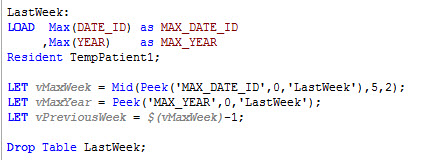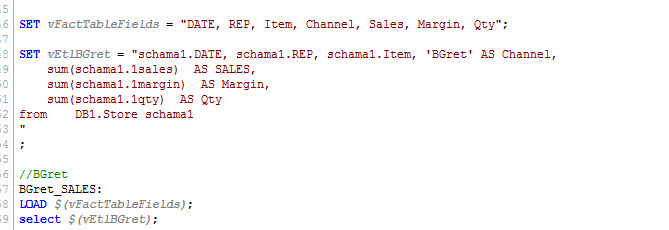New to QlikView

Discussion board where members can get started with QlikView.Not applicable

Peek Functon

Why peek Function work with Let and not with Set ???????????

10 RepliesPartner

Peek Functon

Hi,

Let Function will be Evaluated ur Expression  ,after set Variable

But Set Function Will be Set ur Expression In Variable

Regards

Perumal ANot applicable

Peek Functon

Can anyone explain the peek function with a simple example ?????????????????Not applicable

Re: Peek Functon

The peek function is used to retrieve the contents of a field from a specific table.

Syntax: peek(fieldname [ , row [ , tablename ] ] )

Eg: LET varMinDate = Num(Peek('ExpenseDate', 0, 'Expenses'));

In the above example, 'ExpenseDate is the field and 'Expenses'' is the table from which value is retrieved. The parameter '0' specifies that the value should be retrived from the first row of the ExpenseDate field in the Expenses table. The calculated value is stored in the varMinDate varible and can be used at a later instance in the script.

Similarly, to retrive the value of the last row, '0' can be replaced by -1.

This would be:

Eg: LET varMaxDate = Num(Peek('ExpenseDate', -1, 'Expenses'));

Most often, peek function is used to get the first and last values of a field. However, it can also be used otherwise, of course depending on the scenario.

Hope this helps you.

Regards,

-Khaled.

HighlightedPartner

Re: Peek Functon

Hi Manish..

Let us take an example.

The synatx for peek function is

peek(fieldname [ , row [ , tablename ] ] )

Where fieldname is the field in which you want to apply the function.

Row no---

Row must be an integer. 0 denotes the first record, 1 the second and so on. Negative numbers indicate order from the end of the table. -1 denotes the last record read.

Table name is the name of Table that contain the fields.

Let us understand by an example--

Employee:

EmployeeCode

from

employee.qvd;

now we use the peek function to get the values from the field EmployeeCode from the table Employee

peek('EmployeeCode',-1,Employee);

So in this case we will get the last record from the field.

In case we use 0 than it will return the first record

In case we use 1 than it will return the second record and so on.....

For example ....EmployeeCode =

12

22

45

65

So in case of -1 it will return 65

in case of 1 it will return

22

in case of 0 ,12

regards

KamalNot applicable

Re: Peek Functon

LET statement evaluate the functions included in and after set the variable value.

SET statement is useful to include string constant (also big string elaboration like sql select codes) in a variable.

Look at that useful examples

LET STATEMENTSET STATEMENTPartner

Re: Peek Functon

Hi,

suppose the following table:

Example:

Dim, Value

A, 432

B, 312

C, 423

D, 21

E, 432

F, 432

G, 423

H, 532

I, 423

J, 2312

];

Peek() function allows you to select any record that's been already loaded. This function takes 3 parameters:

1. table name in single quotes
2. the record you want to fetch (0 means the first loaded record, -1 means the last one, -2 the second last one). if no row number is defined, -1 is assumed. Optional parameter
3. the name of the referred table. If no table is defined, the last loaded table will be assumed, Optional parameter.

In the example above, if you define a variable with Peek('Value',0,'Dim') then 432 will be returned by the function:

let vPeek = Peek('Value',0,'Dim');

The variable has to be declared with LET because is something that need to be evaluated. When a variable contains a constant number or a string, then SET can be used. Also it is important to note that Peek() is an inter-record function that can only be used in script,

regards,Not applicable

Re: Peek Functon

SET defines a variable as a literal string --> SET vMath = 2+2 will return "2+2" while LET will return 4.  Just the same, SET will not execute the function, instead, it will return a literal string.  You need to use LET if you want to store an expression in a variable.

Not sure if you can do SET vPeek = \$(=Peek(....) --> Even if you could, not sure if you'd get the same result.  Stick with LET.

New Contributor III

Re: Peek Functon

Hey Guys,

thanks for all the description, but,.... actually i dont see a practical Scenario where i would use peek Funktion.

can someone help me out of that??

ChrisNot applicable

Re: Peek Functon

hi, ı read web servıs, data after take 3 value ın webservıs and joın my functıon.

take all data peek and functıon read all data but ALL ROWS SAVE FIRST READ MY FUNCTON,

let vStartDate = num(date(mid('2015-06-25',1,10 ),'YYYY-MM-DD'));

let vStartTime='00:00:00';

let vEndDate = num(date(mid('2015-06-25',1,10 ),'YYYY-MM-DD'));

FOR i=\$(vStartDate) to \$(vEndDate)

let vDate= date(\$(i),'YYYY-MM-DD');

TEST:

BOOKED ,

CAPACITY,

date(date#(left(DEPDATE,10),'YYYY-MM-DD'),'DD.MM.YYYY') as [Sirena Sefer Tarihi],

Trim(DEPPOINT&'-'&ARRPOINT) AS [Hat],

mid(DEPTIME,12,5) as [Sirena Saat],

mid(DEPTIME,1,10)&mid(DEPTIME,12,5) as [Sirena DateTime],

DETLEVEL,

COMPARTMENT as [Sirena Sınıf],

DEPPOINT,

ARRPOINT,

[DEPPOINT]&''&[ARRPOINT]&''&['A'&PLANETYPE] as KEY,

'A'&PLANETYPE as PLANETYPE,

'KK'&FLIGHTNO as [Sirena Uçuş Numarası]

LET NumRows=NoOfRows('TEST');

FOR i=1 to \$(NumRows)

Let vDEP=Peek('DEPPOINT',\$(i));

Let vARR=Peek('ARRPOINT',\$(i));

Let vACTYPE=Peek('PLANETYPE',\$(i));

join(TEST)